# 2 IV 60 Computer graphics set 7 Basic

• Slides: 392 IV 60 Computer graphics set 7: Basic Geometric Modeling Jack van Wijk TU/eGeometric modeling 1 The world is full of all kind of objects: Trees, people, cars, housed, clouds, rocks, waves, pencil sharpeners, fire, mountains, plants, … How can we describe these, such that they are - easy to enter; - easy to process; - easy to display? Complex problem, HUGE topic!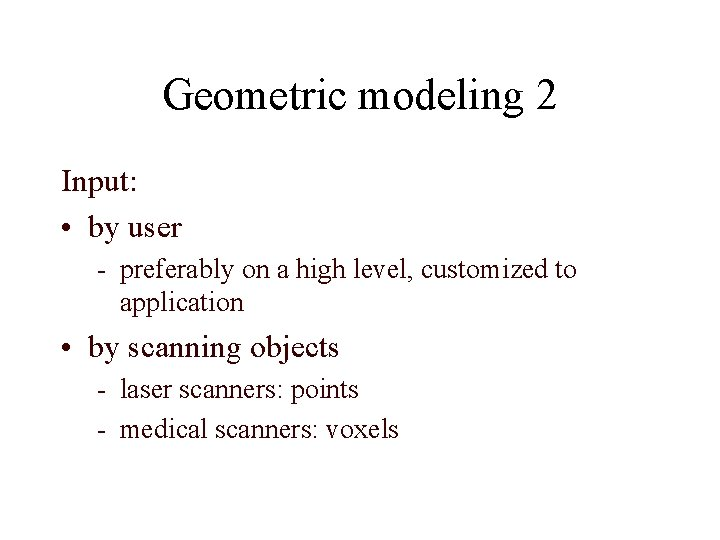Geometric modeling 2 Input: • by user - preferably on a high level, customized to application • by scanning objects - laser scanners: points - medical scanners: voxelsGeometric modeling 3 User Instructions, specifications conversion triangles Scanner 3 D points, voxels conversion graphics pipeline image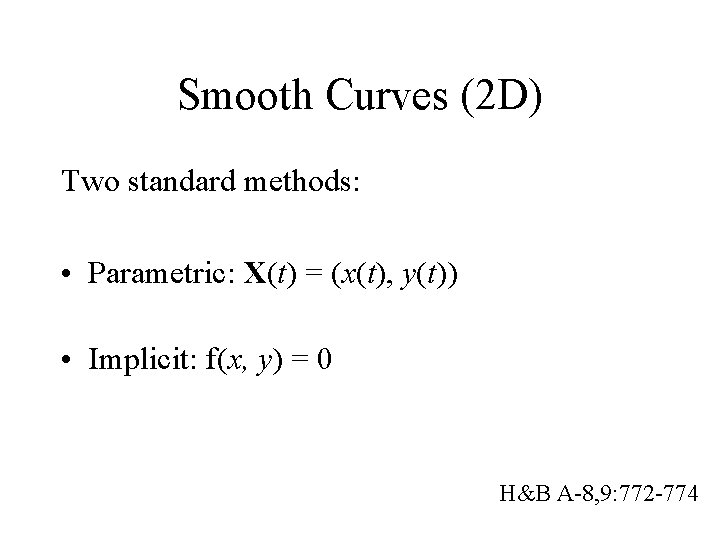Smooth Curves (2 D) Two standard methods: • Parametric: X(t) = (x(t), y(t)) • Implicit: f(x, y) = 0 H&B A-8, 9: 772 -774Parametric description line 1 Given point P and vector V: X(t) = P + Vt y Given two points P en Q: X(t) = P + (Q P)t , or = P(1 t) + Qt Segment: tmin t tmax t Q V P x H&B A-8, 9: 772 -774Parametric description curve • X(t) = (x(t), y(t)) • Drawing a curve in a simple way, approximate with a polyline: Move. To(X(0)); for i : = 1 to N do Line. To(X(i. Dt)); H&B A-8, 9: 772 -774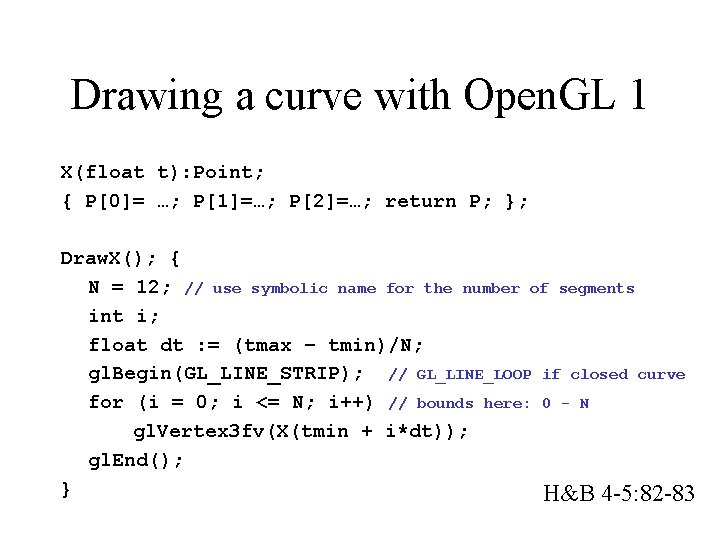Drawing a curve with Open. GL 1 X(float t): Point; { P= …; P=…; P=…; return P; }; Draw. X(); { N = 12; // use symbolic name for the number of segments int i; float dt : = (tmax – tmin)/N; gl. Begin(GL_LINE_STRIP); // GL_LINE_LOOP if closed curve for (i = 0; i <= N; i++) // bounds here: 0 - N gl. Vertex 3 fv(X(tmin + i*dt)); gl. End(); } H&B 4 -5: 82 -83Drawing a curve with Open. GL 2 • Using too few points: – jagged appearance; • Using too many points: – slow, – fat appearance (many segments per pixel). H&B 4 -5: 82 -83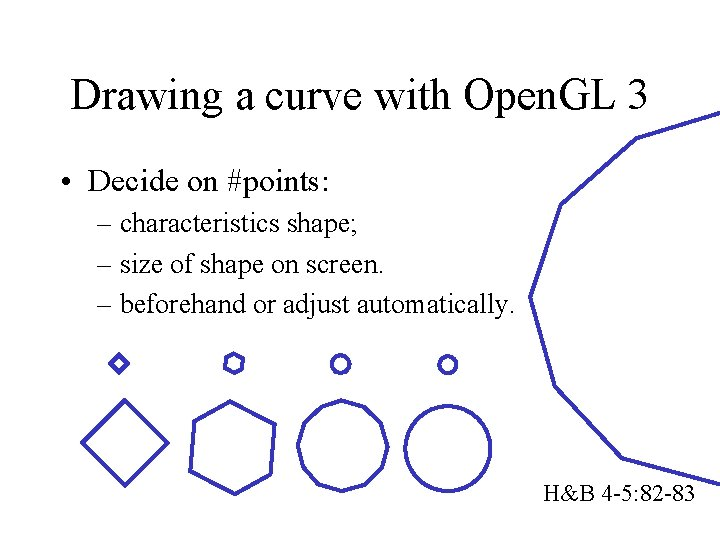Drawing a curve with Open. GL 3 • Decide on #points: – characteristics shape; – size of shape on screen. – beforehand or adjust automatically. H&B 4 -5: 82 -83Implicit description line • (X P). N = 0 with N. V = 0 (N: normal vector) • Also: ax+by+c=0 y N V P x H&B A-8, 9: 772 -774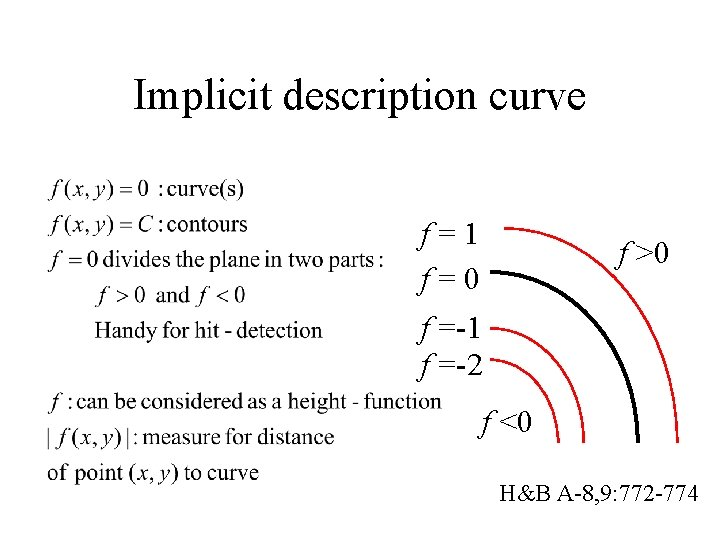Implicit description curve f=1 f=0 f >0 f =-1 f =-2 f <0 H&B A-8, 9: 772 -774Circle y r x H&B A-8, 9: 772 -774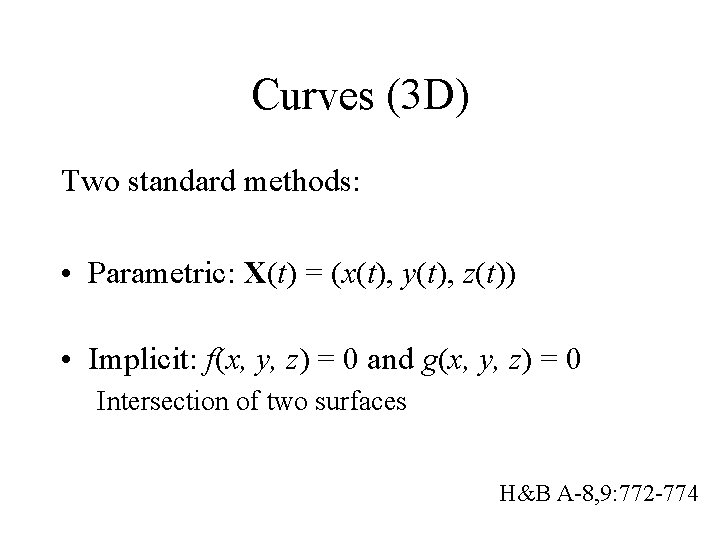Curves (3 D) Two standard methods: • Parametric: X(t) = (x(t), y(t), z(t)) • Implicit: f(x, y, z) = 0 and g(x, y, z) = 0 Intersection of two surfaces H&B A-8, 9: 772 -774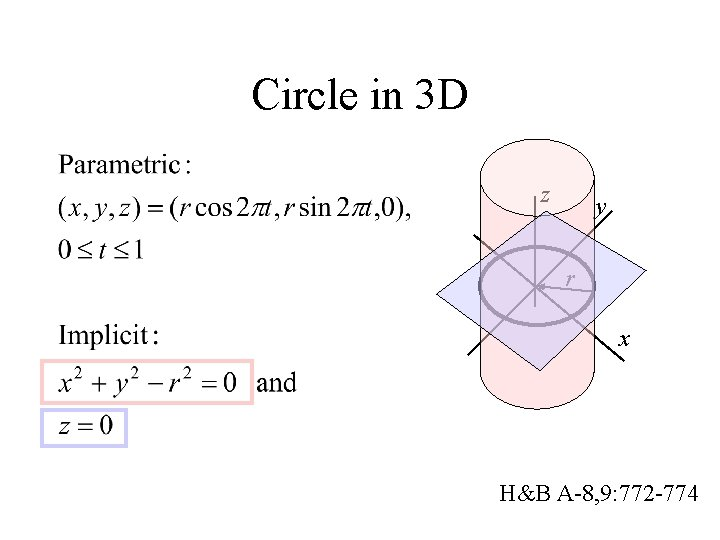Circle in 3 D z y r x H&B A-8, 9: 772 -774Surfaces • Polyhedra • Parametric surfaces • Implicit surfaces H&B A-8, 9: 772 -774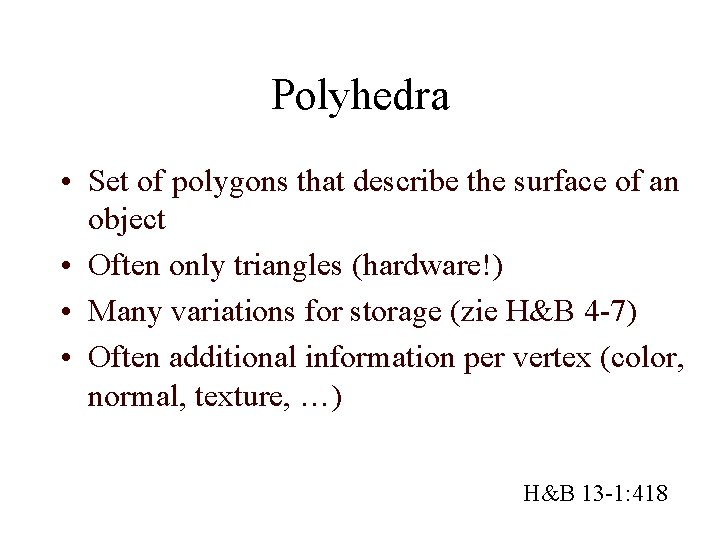Polyhedra • Set of polygons that describe the surface of an object • Often only triangles (hardware!) • Many variations for storage (zie H&B 4 -7) • Often additional information per vertex (color, normal, texture, …) H&B 13 -1: 418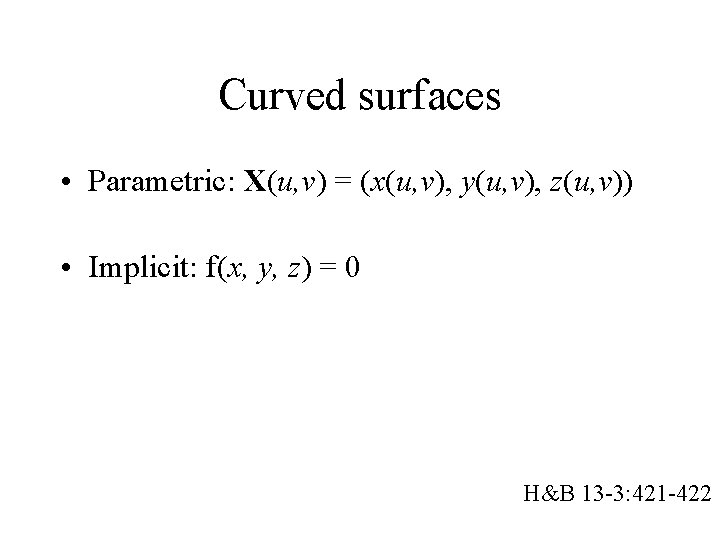Curved surfaces • Parametric: X(u, v) = (x(u, v), y(u, v), z(u, v)) • Implicit: f(x, y, z) = 0 H&B 13 -3: 421 -422Sphere 1 z y x u constant, v varies v constant, u varies H&B 13 -4: 422 -424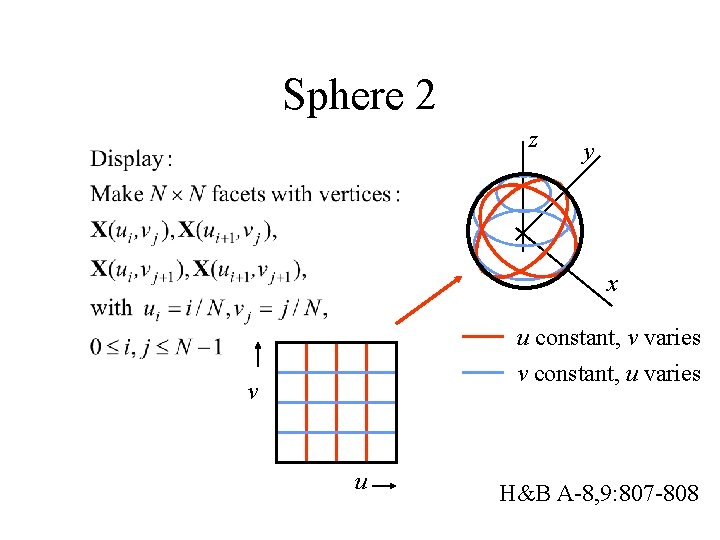Sphere 2 z y x u constant, v varies v constant, u varies v u H&B A-8, 9: 807 -808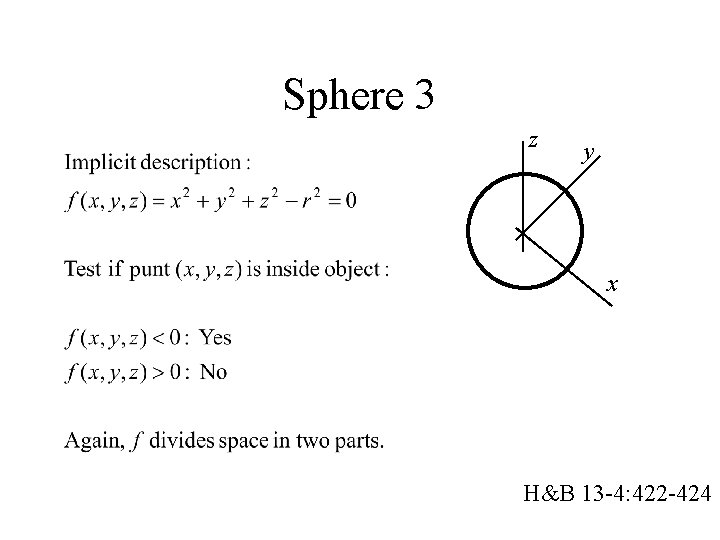Sphere 3 z y x H&B 13 -4: 422 -424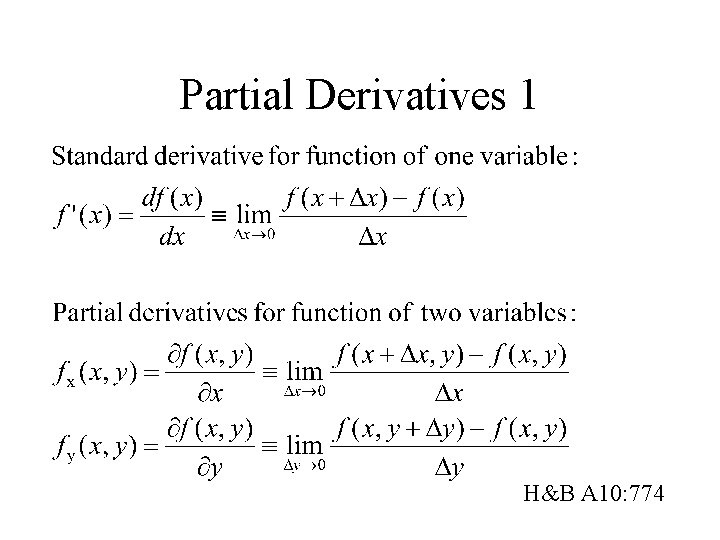Partial Derivatives 1 H&B A 10: 774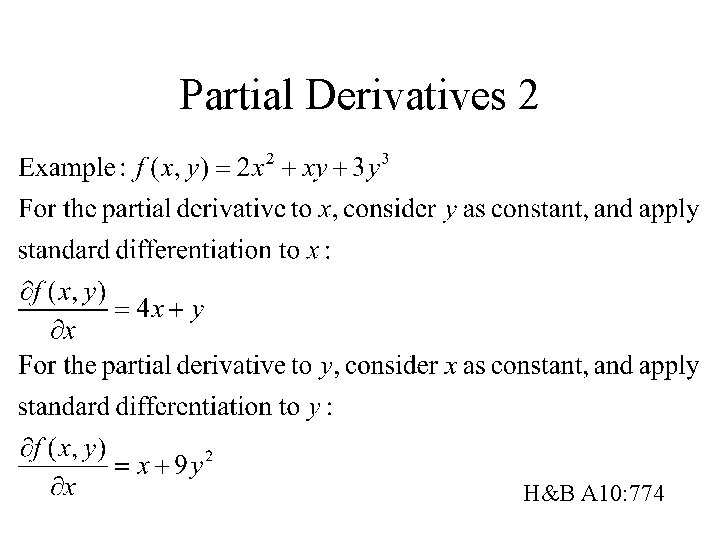Partial Derivatives 2 H&B A 10: 774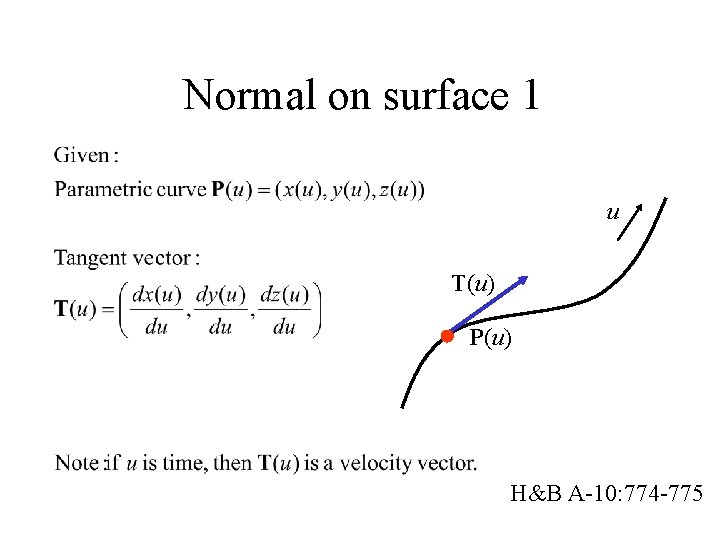Normal on surface 1 u T(u) P(u) H&B A-10: 774 -775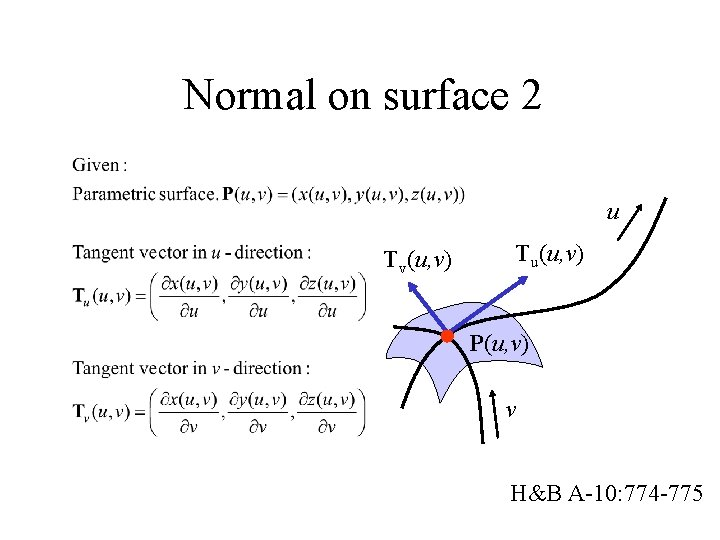Normal on surface 2 u Tv(u, v) Tu(u, v) P(u, v) v H&B A-10: 774 -775Normal on surface 3 u N Tv(u, v) Tu(u, v) P(u, v) v H&B A-10: 774 -775Normal on surface 4 z Tv v N Tu x y u H&B A-10: 774 -775Normal on surface 5 H&B A-10: 774 -775Normal on surface 6 N(x, y, z) f(x, y, z)=0 H&B A-10: 774 -775Normal on surface 7 f >0 f=0 f <0 H&B A-10: 774 -775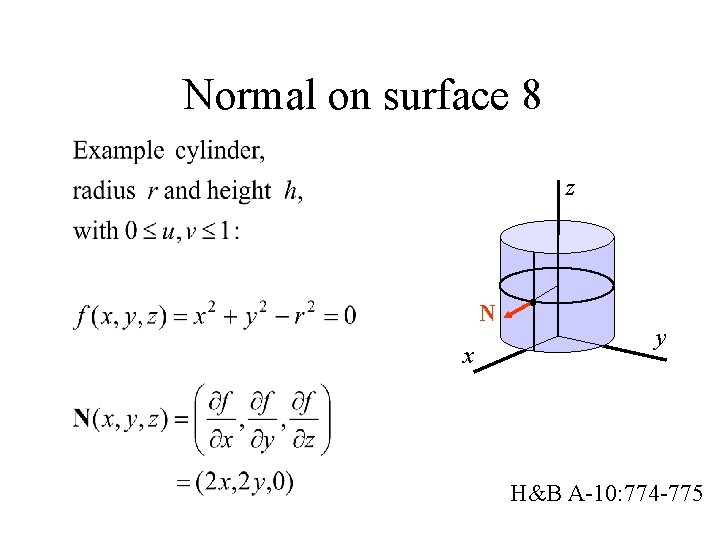Normal on surface 8 z N x y H&B A-10: 774 -775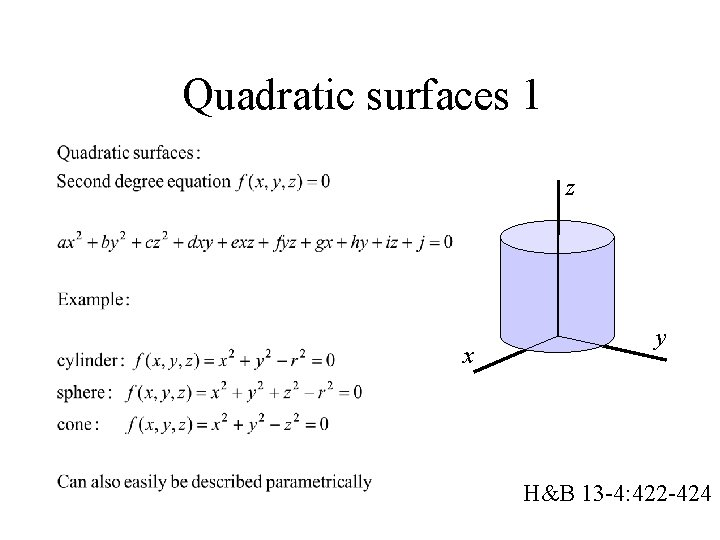Quadratic surfaces 1 z x y H&B 13 -4: 422 -424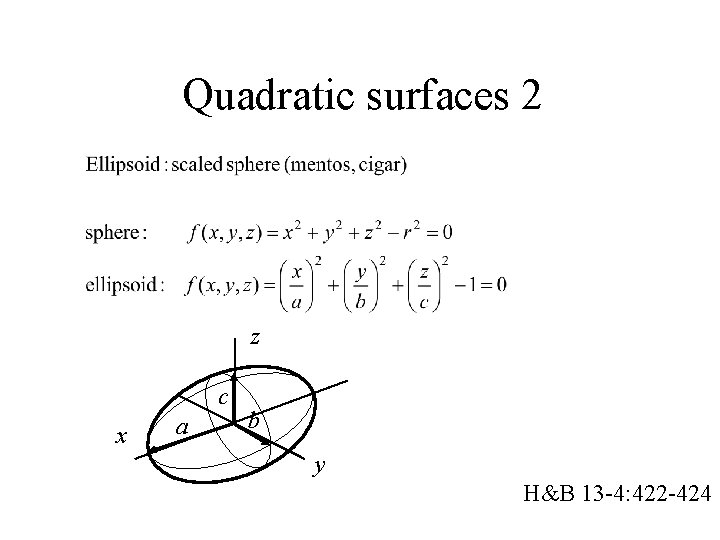Quadratic surfaces 2 z c x a b y H&B 13 -4: 422 -424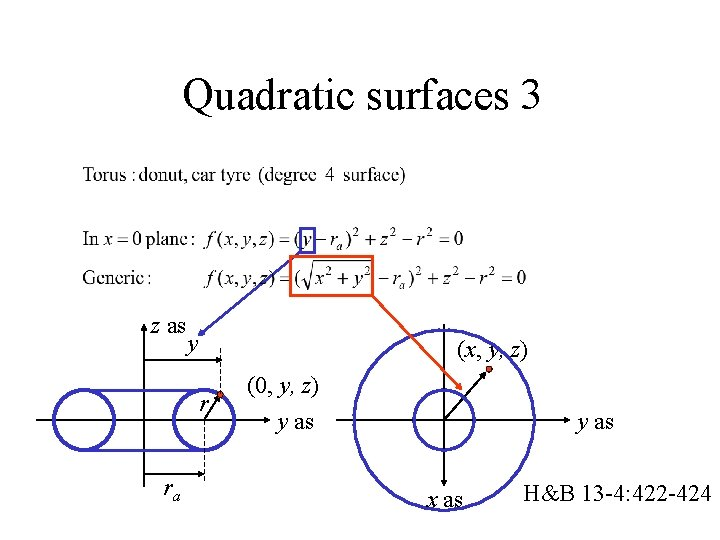Quadratic surfaces 3 z as y (x, y, z) r ra (0, y, z) y as x as H&B 13 -4: 422 -424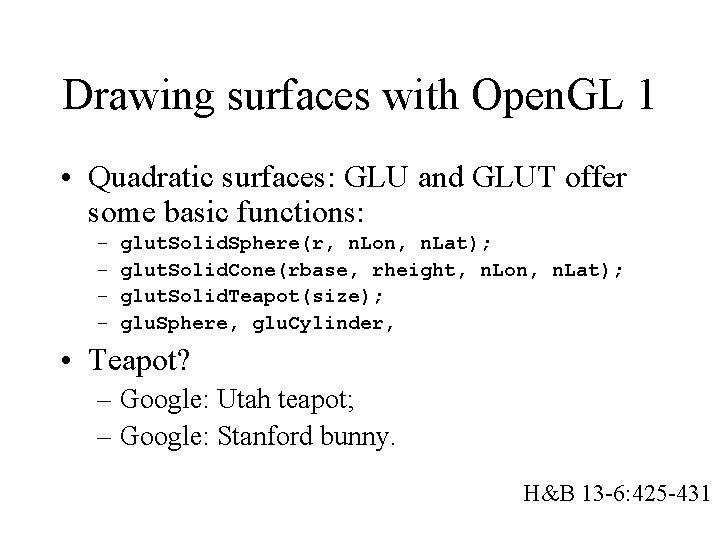Drawing surfaces with Open. GL 1 • Quadratic surfaces: GLU and GLUT offer some basic functions: – – glut. Solid. Sphere(r, n. Lon, n. Lat); glut. Solid. Cone(rbase, rheight, n. Lon, n. Lat); glut. Solid. Teapot(size); glu. Sphere, glu. Cylinder, • Teapot? – Google: Utah teapot; – Google: Stanford bunny. H&B 13 -6: 425 -431Drawing surfaces with Open. GL 1 • Quadratic surfaces: GLU and GLUT offer some basic functions: – – glut. Solid. Sphere(r, n. Lon, n. Lat); glut. Solid. Cone(rbase, rheight, n. Lon, n. Lat); glut. Solid. Teapot(size); glu. Sphere, glu. Cylinder, • Alternative: Define your own, custom versions, based on standard way to render parametric surfaces. H&B 13 -6: 425 -431Drawing surfaces with Open. GL 2 X(float u, v): Point; { P= …; P=…; P=…; return P; }; Draw. X(); { NU, NV = 12; // use symbolic names int i, j; float du : = (umax – umin)/NU; float dv : = (vmax – vmin)/NV; for numbers of points again for (j = 0; j < NV; j++) { gl. Begin(GL_QUAD_STRIP); for (i = 0; i <= NU; j++) { gl. Vertex 3 fv(X(umin + i*du, vmin + j*dv)); gl. Vertex 3 fv(X(umin + i*du, vmin + (j+1)*dv)); } gl. End(); }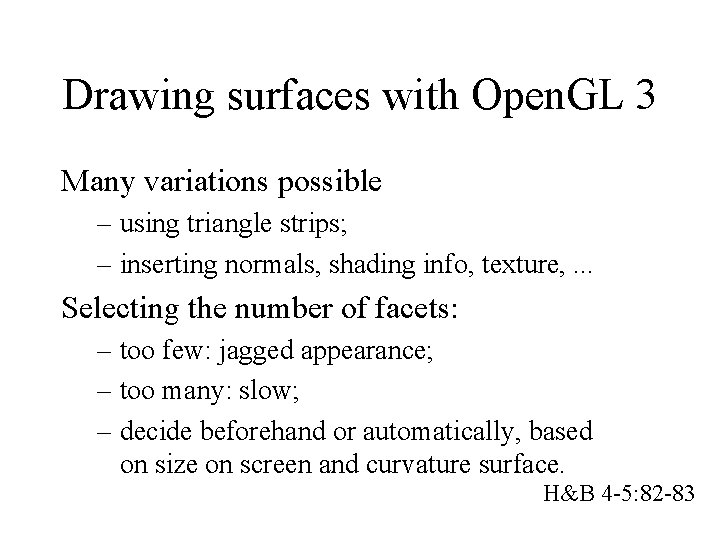Drawing surfaces with Open. GL 3 Many variations possible – using triangle strips; – inserting normals, shading info, texture, . . . Selecting the number of facets: – too few: jagged appearance; – too many: slow; – decide beforehand or automatically, based on size on screen and curvature surface. H&B 4 -5: 82 -83Next… • We now know how to define basic objects. • Stay tuned for splines: arbitrary curves and curved surfaces. • But first: let’s consider illumination and shading.